# how to map (1V - 10V) to (1.6V - 3.2V) for voltage regulation using TL494

#### silvi09

Joined Sep 26, 2021
11
I am trying to design the given circuit where pin 1 is the non inverting terminal of comparator where I want to use a ref voltage of 1V - 10V but IC TL494 is accepting a voltage of 1.6V to 3.2V only for generating a pwm ....and at pin 2 m trying to give an o/p voltage feedback which varies from 10V - 100V through resistor divider circuit.. So how could i map (1- 10V) to (1.6 to 3.2)V through resistor divider circuit..

#### ericgibbs

Joined Jan 29, 2010
15,487
hi silvi,
The problem with a resistive divider, is getting the 1V up to 1.6V

Do you have any OPA's on you work bench.?
E

#### Papabravo

Joined Feb 24, 2006
18,400
The first step is to work out the equation of the linear transformation you wish to implement. You have two points and the slope, the equation is straight forward:
$$(x_0,y_0)=(1,1.6)$$
$$(x_1,y_1)=(10,3.2)$$
$$m=\cfrac{(y_1-y_0)}{(x_1-x_0)}=\cfrac{3.2-1.6}{10-1}\approx0.1778$$
Using the slope, m, and one of the points, we can write:
$$(y-y_0)=m(x-x_0)\;\text{ ; point slope form of a line}$$
$$(y-1.6)=0.1778(x-1)$$
$$y=0.1778x+1.4222$$
Since the slope is less than 1, you are going to need two operational amplifiers in an inverting configuration. Do you know how to implement the equation of a line in this fashion?

Edit: I did get it wrong, corrected above
So, with the correct linear function the 2-opamp single package solution is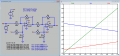Edit2: If there is any advantage to this method it is the ability to control the slope and the offset independently.
V(o) = 2*V(vref) - V(a), or V(vref) = (V(o) + V(a))/2

Last edited:
•#### BobTPH

Joined Jun 5, 2013
4,884
Rewrite it as

y = (x + 2.3884) * .4722

And you can see how to do it with one op amp.

Bob

#### Irving

Joined Jan 30, 2016
2,662
y=0.4722x+1.1278
x = 1, y = 1.6
x = 10, y = 5.85, not 3.2 as requested....

Should be (3.2 - 1.6)/(10 - 1) = 1.6/9 = 0.1778 (slope)

Intercept = 1.6 - 0.1778 = 1.4222

y = 0.1778 x + 1.4222

x = 1, y = 0.1778 + 1.4222 = 1.6
x = 10, y = 1.778 + 1.4222 = 3.2

Easiest way to do this is using a cheap instrumentation amplifier. AD620, AD623, LT1168 etc... The reference input sets the intercept voltage, and a simple resistive divider sets the slope. Here I've split the bottom resistor of your 10:1 divider to give the additional divider. 1% resistors are good enough in standard values for this....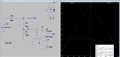•dcbingaman and Papabravo

#### ericgibbs

Joined Jan 29, 2010
15,487

•Papabravo

#### crutschow

Joined Mar 14, 2008
29,755
Eric,
I noticed you used a resistor for a pot.
Attached are the pot files I use, if interested.

#### Attachments

• 758 bytes Views: 5
• 193 bytes Views: 4
•ericgibbs

#### MrAl

Joined Jun 17, 2014
8,972
Hello there,

Here is a procedure for calculating the required resistor values. The circuit is similar to Eric's because if we try to do a passive circuit we get no solution unless we can implement negative resistance, and since we usually dont want to do that we have to incorporate some sort of gain into the circuit. The procedure therefore has to use at least one op amp although there may be other possibilities for the gain part of it.

The entire schematic and procedure is shown in the attachment so it can be all in one place in one single file. The crux of the calculations involve calculating R1 and R2 so i start with that.
Note the input resistor values reflect resistor ratios not absolute resistances as that simplifies the calculations a bit.
The image is a little large so i wont make it appear inline.

#### MrAl

Joined Jun 17, 2014
8,972
Hello again,

Oh there is one resistor only solution but unfortunately it requires an 8 volt floating DC source.
You can change it to an 8 volt grounded source, but then the input voltage Vin has to be floating, which might be possible.
To get the 8v grounded source, you can use a 10 volt source with a low impedance divider.

Here is the circuit...and again it is 1 to 10 volt input and 1.6 to 3.2 volt output.
Again scale R1 and R2 as needed to match impedance needs with some interaction between input and output impedances.
So if you make R2=1k then R1=4.625k for example. Output impedance is then 822.2 Ohms and input impedance is 5.625k with nothing connected to output, and a bit lower if there is.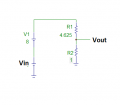#### crutschow

Joined Mar 14, 2008
29,755
It can be done with 3 resistors and a grounded reference voltage with no op amp needed.

My method of calculation was to initially determine where the input and output voltage would be equal (≈1.7295V).
At that point there's no current through R3, and you can calculate the ratio of R1 and R2 to give 1.7295V from the 5V reference.
Then you can set the input to 1V and calculate the value of R3 to give an output voltage of 1.6V (or alternate set the input to 10V and select R3 to give 3.2V output).
(I studiously try to avoid any equations with more than one unknown at a time).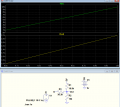#### MrAl

Joined Jun 17, 2014
8,972
It can be done with 3 resistors and a grounded reference voltage with no op amp needed.

My method of calculation was to initially determine where the input and output voltage would be equal (≈1.7295V).
At that point there's no current through R3, and you can calculate the ratio of R1 and R2 to give 1.7295V from the 5V reference.
Then you can set the input to 1V and calculate the value of R3 to give an output voltage of 1.6V (or alternate set the input to 10V and select R3 to give 3.2V output).
(I studiously try to avoid any equations with more than one unknown at a time).View attachment 266893
Hi,

Yeah you beat me by an hour i just found this also.
Here's my drawing...
Again the resistors are in terms of ratios not absolute values, so you can set the impedances but they are somewhat interactive.

With reference to the DC offset input E2, the calculations are as follows:
[R1=(37*R3)/8-(8*R3)/E2,R2=(37*E2*R3)/64-R3]
and if you set R3=1 again you get the ratios. To convert to actual resistor values, just multiply all by the same constant, such as 1000 or something like that. So first set E2 to whatever stable DC voltage you have available.

#### Attachments

•crutschow

#### crutschow

Joined Mar 14, 2008
29,755
So first set E2 to whatever stable DC voltage you have available.
What would be the minimum voltage for that?

I tried your calculations with a 2.5V reference, but the output was not correct (top sim below).
I double checked my calculations with the same result.
Using values from my calculation the results are correct (bottom sim).
Any thoughts on that?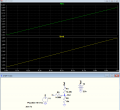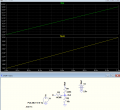Last edited:

#### Irving

Joined Jan 30, 2016
2,662
Hmmm ... going back to the initial post, I'm not sure the OP has the right requirement. The TL494 does indeed have a 1.6 - 3.2v limit on the input but that's only for the control range of the PWM. The actual central feedback voltage for a required output voltage should be 2.5v. The TL494 is primarily designed for fixed output voltage. In a non-isolated output that would be a direct tapped connection from the output voltage to the error amp (so 5v is dropped to 2.5v by a simple 2:1 voltage divider as shown in the datasheet). The isolated step-up converter with a 10 - 100v output needs a different approach. The feedback needs to be on the output side of the isolation transformer with an opto-coupler providing a simple on/off switch to the PWM as per this snippet from TI application note "Isolated Multiple Output Flyback Converter Design Using TL494". Here the TL431 (U2) samples the output voltage through R1/R2 and switches the LED of the opto-coupler on/off - this gates the PWM directly.

So for a 10 - 100v output range, the R1/R2 ratio needs to vary from 3:1 to 39:1 to maintain the voltage at the reference input of U2 at 2.5v. That's a very different problem, not aided by the TL494 being a very old design which isn't best suited for a variable output PSU. Because this feedback ratio directly impacts the loop gain, stability and regulation will suffer at higher output voltages.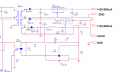#### MrAl

Joined Jun 17, 2014
8,972
What would be the minimum voltage for that?

I tried your calculations with a 2.5V reference, but the output was not correct (top sim below).
I double checked my calculations with the same result.
Using values from my calculation the results are correct (bottom sim).
Any thoughts on that?

View attachment 266955

View attachment 266956
Hello again,

I see you switched resistor enumerations, and that's not too much of a problem i guess, but it looks like you calculated the values slightly off.
If i posted the wrong formulas i'll post here again:
[R1=37/8-8/E2,R2=(37*E2)/64-1]
and with E2=2.5 and with scaling factor of 10k i get:
R1=14250.0
R2=4453.125
R3=10000
and this seems to work but you can try it yourself.

Since we cant have either the input source Vin or the DC offset source connect directly to the output (the output could then never change) when either R1 or R2 comes out to zero (0 Ohms) that would not work, so anything above the value(s) that cause either to go to zero unless we accept negative resistances and then anything below the zero value(s).
As it turns out, both R1 and R2 got to zero when E2=64/37 so anything above that would work in theory, but of course we have to assign reasonable values to both and your scaling factor of 10k sounds very good.

So try that calculation again and swap resistor enumerations as you did before and see what you get.

You know what is funny is this is the first network i looked at when i first saw this thread, but i did something wrong in the calculation so it looked like it didnt work. Only when i went back and looked at it again i found out the mistake.

There is one slight drawback though that plagues all resistor dividers, and that is the input and output impedances can not be set independent of one another. I think it takes an op amp to do that, but could it be done with a balanced resistor network? We'd have to look into that.